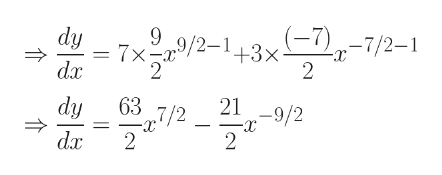derivative of 7x^4sqrt(x)+3/(x^3sqrt(x))

Question

derivative of 7x^4sqrt(x)+3/(x^3sqrt(x))

Step 1

Let's call the given expression as y.

Also recall the rule of exponents: Pa x Pb = Pa+b

Please see the white board.

Step 2

Recall the famous rule of differentiation: d(xn) / dx = nxn-1...help_outlineImage Transcriptionclose9 7x dy 2-143x -7/2-1 dx 219/2 63 7/2 dy 2 2 da fullscreen

Want to see the full answer?

See Solution

Want to see this answer and more?

Our solutions are written by experts, many with advanced degrees, and available 24/7

See Solution
Tagged in

Derivative